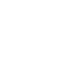Research in Pairs
Research Community
Natasha Samko
PhD

Professor of Mathematics, UiT The Arctic University of Norway

1
Research interests
operator theory, integral equations, function spaces
2
Projects supported by the Regional Mathematical Center
Integrability properties of integral transforms via Morrey spaces
We show that integrability properties of integral transforms with kernel depending on the product of arguments (which include in particular, popular Laplace, Hankel, Mittag-Leffler transforms and various others) are better described in terms of Morrey spaces than in terms of Lebesgue spaces. Mapping properties of integral transforms of such a type in Lebesgue spaces, including weight setting, are known. We discover that local weighted Morrey and complementary Morrey spaces are very appropriate spaces for describing integrability properties of such transforms. More precisely, we show that under certain natural assumptions on the kernel, transforms under consideration act from local weighted Morrey space to a weighted complementary Morrey space and vice versa, where an interplay between behavior of functions and their transforms at the origin and infinity is transparent. In case of multidimensional integral transforms, for this goal we introduce and use anisotropic mixed norm Morrey and complementary Morrey spaces.
Natasha Samko Integrability properties of integral transforms via Morrey spaces // Fractional Calculus and Applied Analysis, Vol. 23: N 5. https://doi.org/10.1515/fca-2020-0064

On weighted Fourier inequalities - some new scales of equivalent conditions
For Lebesgue spaces on Rn, we study two-weight p → q-inequalities for Fourier transform. Some sufficient conditions on weights for such inequalities are known for special ranges of parameters p and q. In the same ranges of parameters we show, that in every case each of those conditions can be replaced by infinitely many conditions, even by continuous scales of conditions. We also derive some new such characterizations concerning the Fourier transform in weighted Lorentz spaces.
A. Kufner, L.E. Persson, and N. Samko. On weighted Fourier inequalities - some new scales of equivalent conditions //  J. Math. Inequal. Volume 15, Number 2 (2021), 879–898. dx.doi.org/10.7153/jmi-2021-15-61
In Focus